# 用浏览器训练Tensorflow.js模型的18个技巧（上）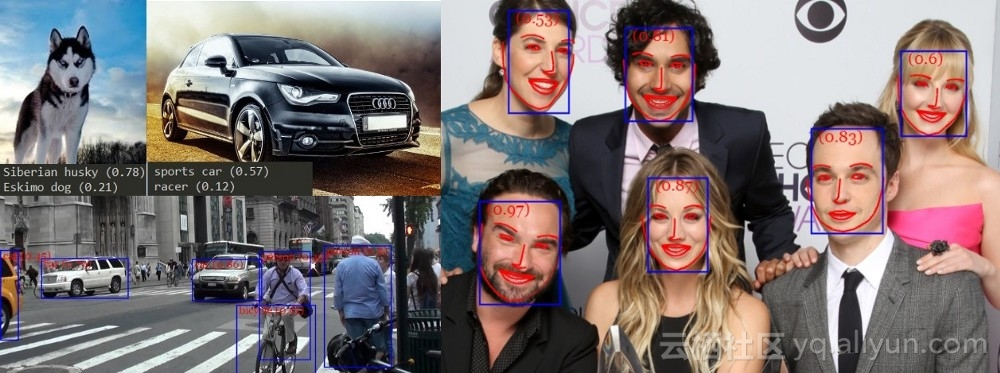Web训练高效的深度学模型### 网络架构

#### 2.采用深度可分的卷积！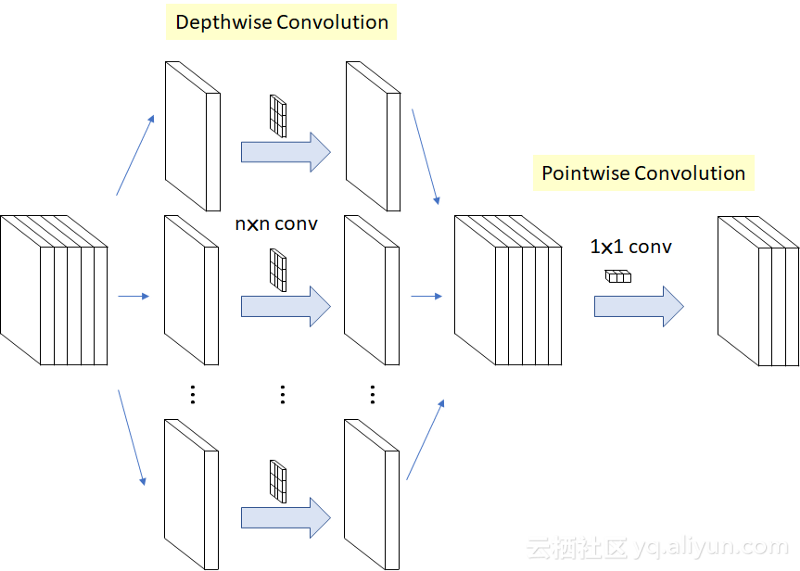MobileNetXception采用了深度可分离卷积的思想，你可以在MobileNetPoseNet的tensorflow.js模型中找到它们。深度可分离卷积是否导致模型不太准确可能是一个公开的辩论，但根据我的经验，它们绝对是网络模型的方式。

export type ConvParams = {
filter: tf.Tensor4D
bias: tf.Tensor1D
}
export function convLayer(
x: tf.Tensor4D,
params: ConvParams,
stride: [number, number],
): tf.Tensor4D {
return tf.tidy(() => {
let out = tf.conv2d(x, params.filter, stride, padding)
return out
})
}

export type SeparableConvParams = {
depthwise_filter: tf.Tensor4D
pointwise_filter: tf.Tensor4D
bias: tf.Tensor1D
}
export function depthwiseSeparableConv(
x: tf.Tensor4D,
params: SeparableConvParams,
stride: [number, number],
): tf.Tensor4D {
return tf.tidy(() => {
let out = tf.separableConv2d(x, params.depthwise_filter: tf.Tensor4D, params.pointwise_filter, stride, padding)
return out
})
}

#### 3.跳过连接（Skip connections）和密集连接（Densely Connected）的块

ResNet和DenseNet中使用的跳过连接允许它们构建更深层的体系结构，同时减轻梯度消失问题。我们所要做的就是在应用激活函数之前，将先前层的输出添加到位于网络中更深层的层输入中：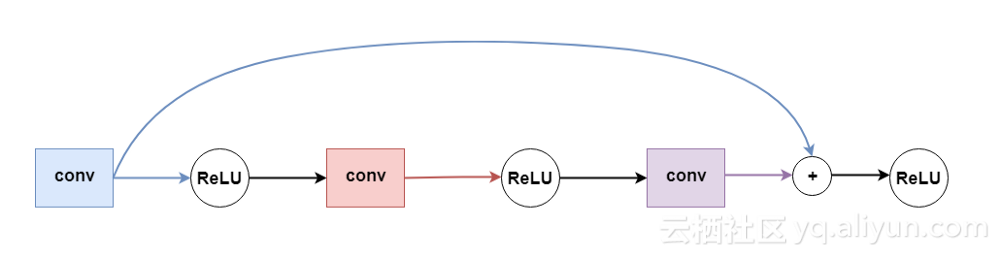4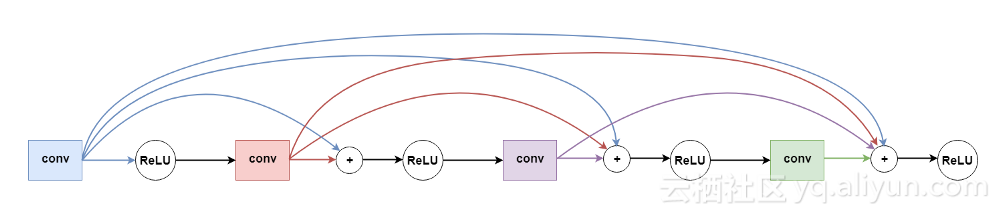5

export type DenseBlock4Params = {
conv0: SeparableConvParams | ConvParams
conv1: SeparableConvParams
conv2: SeparableConvParams
conv3: SeparableConvParams
}

export function denseBlock4(
x: tf.Tensor4D,
denseBlockParams: DenseBlock4Params,
isFirstLayer: boolean = false
): tf.Tensor4D {
return tf.tidy(() => {
const out0 = isFirstLayer
? convLayer(x, denseBlockParams.conv0 as ConvParams, [2, 2], 'same')
: depthwiseSeparableConv(x, denseBlockParams.conv0 as SeparableConvParams, [2, 2], 'same')
as tf.Tensor4D
const in1 = tf.relu(out0) as tf.Tensor4D
const out1 = depthwiseSeparableConv(in1, denseBlockParams.conv1, [1, 1], 'same')

// first join
const in2 = tf.relu(tf.add(out0, out1)) as tf.Tensor4D
const out2 = depthwiseSeparableConv(in2, denseBlockParams.conv2, [1, 1], 'same')

// second join
const out3 = depthwiseSeparableConv(in3, denseBlockParams.conv3, [1, 1], 'same')

// final join
})
}

#### 4.使用ReLU类型激活函数！

export function leakyRelu(x: tf.Tensor, epsilon: number) {
return tf.tidy(() => {
const min = tf.mul(x, tf.scalar(epsilon))
return tf.maximum(x, min)
})
}

export function relu6(x: tf.Tensor) {
return tf.clipByValue(x, 0, 6)
}

### 训练

const optimizer = tf.train.adam(0.001)

#### 6.调整学习率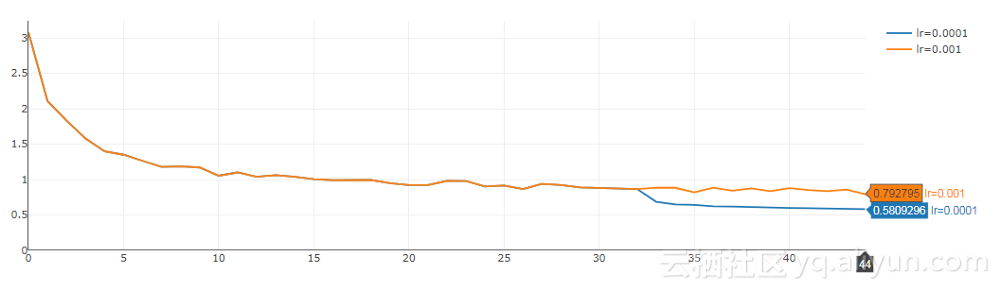6

#### 7.权重初始化

const initializer = tf.initializers.glorotNormal()
const depthwise_filter = initializer.apply([3, 3, 32, 1])
const pointwise_filter = initializer.apply([1, 1, 32, 64])
const bias = tf.zeros()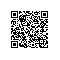使用钉钉扫一扫加入圈子
+ 订阅